# R Plotting

## Plot

The `plot()` function is used to draw points (markers) in a diagram.

The function takes parameters for specifying points in the diagram.

Parameter 1 specifies points on the x-axis.

Parameter 2 specifies points on the y-axis.

At its simplest, you can use the `plot()` function to plot two numbers against each other:

### Example

Draw one point in the diagram, at position (1) and position (3):

plot(1, 3)

Result: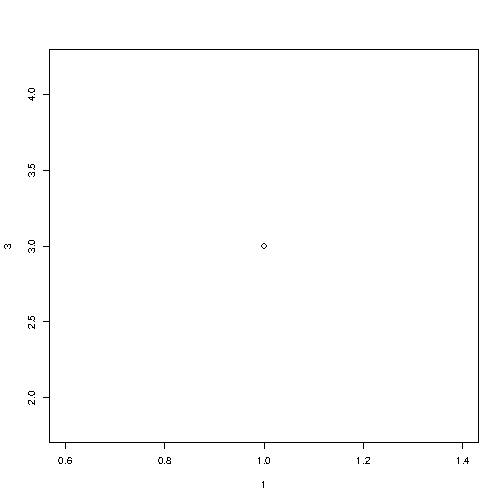Try it Yourself »

To draw more points, use vectors:

### Example

Draw two points in the diagram, one at position (1, 3) and one in position (8, 10):

plot(c(1, 8), c(3, 10))

Result: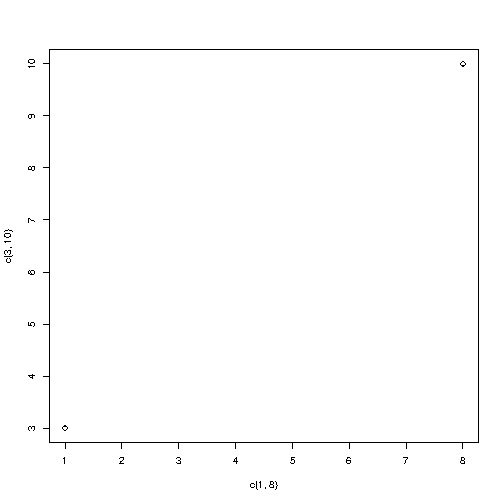Try it Yourself »

## Multiple Points

You can plot as many points as you like, just make sure you have the same number of points in both axis:

### Example

plot(c(1, 2, 3, 4, 5), c(3, 7, 8, 9, 12))

Result: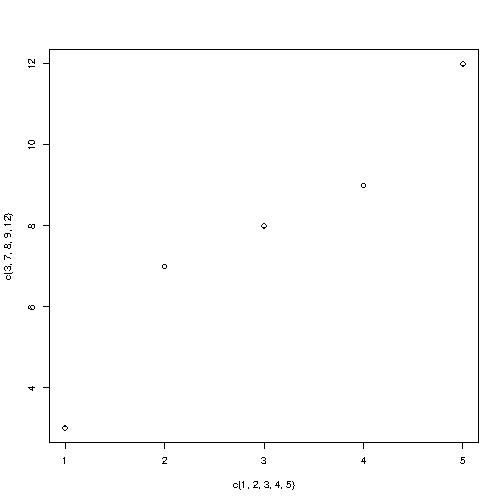Try it Yourself »

For better organization, when you have many values, it is better to use variables:

### Example

x <- c(1, 2, 3, 4, 5)
y <- c(3, 7, 8, 9, 12)

plot(x, y)

Result:Try it Yourself »

## Sequences of Points

If you want to draw dots in a sequence, on both the x-axis and the y-axis, use the `:` operator:

### Example

plot(1:10)

Result: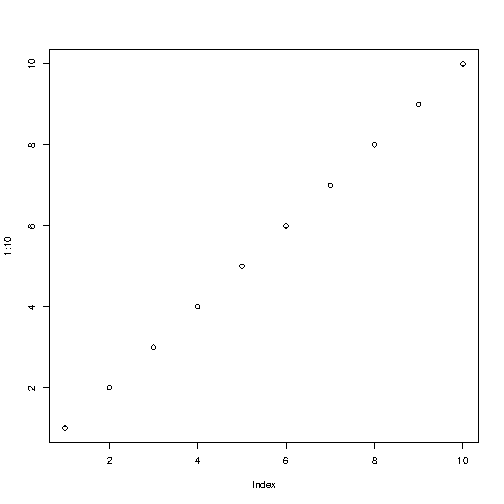Try it Yourself »

## Draw a Line

The `plot()` function also takes a `type` parameter with the value `l` to draw a line to connect all the points in the diagram:

### Example

plot(1:10, type="l")

Result: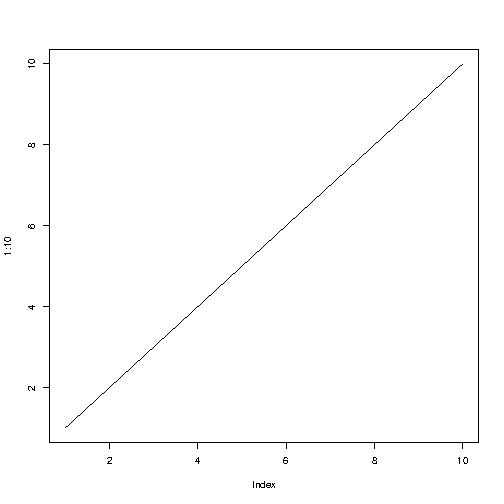Try it Yourself »

## Plot Labels

The `plot()` function also accept other parameters, such as `main`, `xlab` and `ylab` if you want to customize the graph with a main title and different labels for the x and y-axis:

### Example

plot(1:10, main="My Graph", xlab="The x-axis", ylab="The y axis")

Result: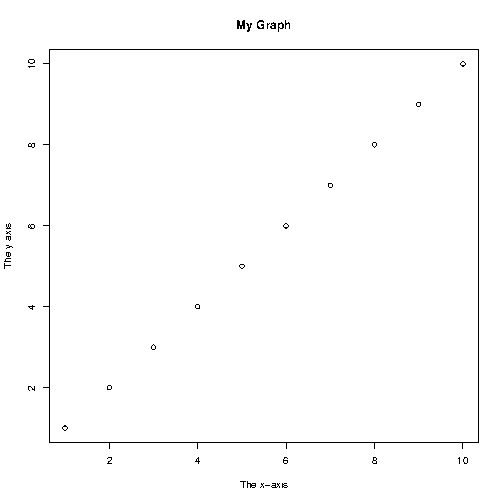Try it Yourself »

## Graph Appearance

There are many other parameters you can use to change the appearance of the points.

### Colors

Use `col="color"` to add a color to the points:

### Example

plot(1:10, col="red")

Result: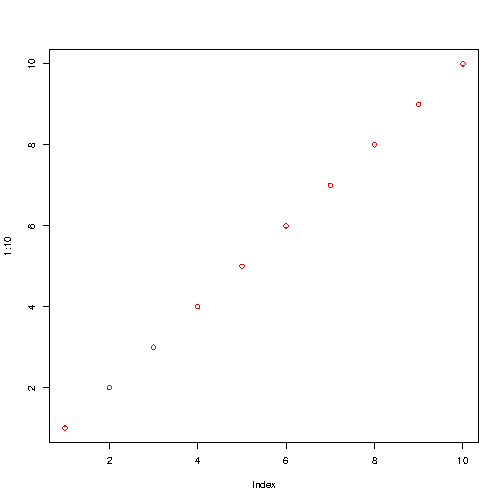Try it Yourself »

### Size

Use `cex=number` to change the size of the points (`1` is default, while `0.5` means 50% smaller, and `2` means 100% larger):

### Example

plot(1:10, cex=2)

Result:Try it Yourself »

### Point Shape

Use `pch` with a value from 0 to 25 to change the point shape format:

### Example

plot(1:10, pch=25, cex=2)

Result:Try it Yourself »

The values of the `pch` parameter ranges from 0 to 25, which means that we can choose up to 26 different types of point shapes: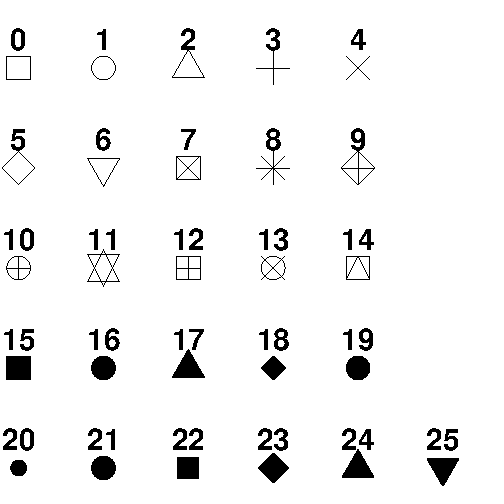W3Schools is optimized for learning and training. Examples might be simplified to improve reading and learning. Tutorials, references, and examples are constantly reviewed to avoid errors, but we cannot warrant full correctness of all content. While using W3Schools, you agree to have read and accepted our terms of use, cookie and privacy policy.